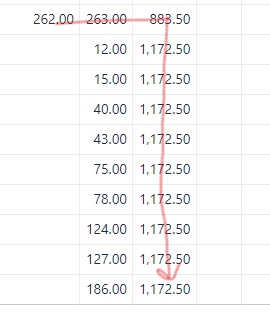# Fill empty cell with last value

Hi,

I have calculated measure that is showing numbers until end of the defined period. I need help with additional measure that will take last value of measure mentioned and fill empty cells into future, similar to cumulative.

In this case I want 262 in third columnCurrently I’m using cumulative sum formula but I don’t know how to modify it to sum only last cell (I have the exact date of last value in first column)

``````case WHEN
DateBetween(
[Time].CurrentHierarchyMember.StartDate,
[Measures].[Enter 2nd date],
[Time].CurrentHierarchyMember.NextStartDate
)
THEN
Sum(
PreviousPeriods([Time].CurrentHierarchyMember),
[Measures].[prediction])
end
``````

Hi @TaraWin ,
If you wish to generate your future values based on the last entry, you can try this formula (change measure “Issues with due date” to your measure name). The formula works like this - if the value itself is empty, then it will return measure for the last member from the VisibleRowsSet(), otherwise, it will return the measure itself:

``````IIF(
IsEmpty([Measures].[Issues with due date]),
((Tail(Filter(
VisibleRowsSet(),
[Measures].[Issues created]>0
),1)).Item(0).Item(0),
[Measures].[Issues with due date]),
[Measures].[Issues with due date]
)
``````

In the report:

best,
Gerda // support@eazyBI.com

Hi @gerda.grantina , I want to thank you for this solution. You saved me a lot of time, thanks again!

1 Like